# Linear function, graph of a linear function

Definition: a Linear function is a function of the form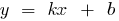where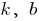is some number

## Properties of linear functions

1. Scope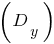2.3. Multiple value4. when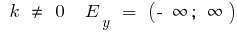when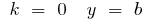5. Parity, odd parity
6. if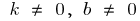the function is neither even nor odd

in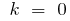— steamroom

ifand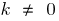is odd

7. The point of intersection with the axes
8. at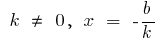the point of intersection with the axisthen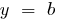straight and parallel to the axis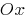at a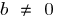and coincides with the axiswhen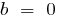9. Continuity and differentiability
10. A linear function is continuous and diferencian on the entire number line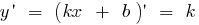11. The increase and decrease of
12. when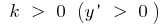a function is increasing on all number line

when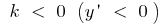a function is decreasing on the entire number line

when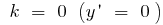a function became

13. The graph of a linear function is always a straight, tangent of slope angle of this straight line to the axis

atthe point of intersection with the axis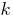— the angular coefficient of the straightwhen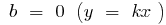is a straight line passing through the origin

when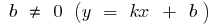is a straight line, not passing through the origin

## Graphs of linear functions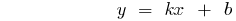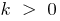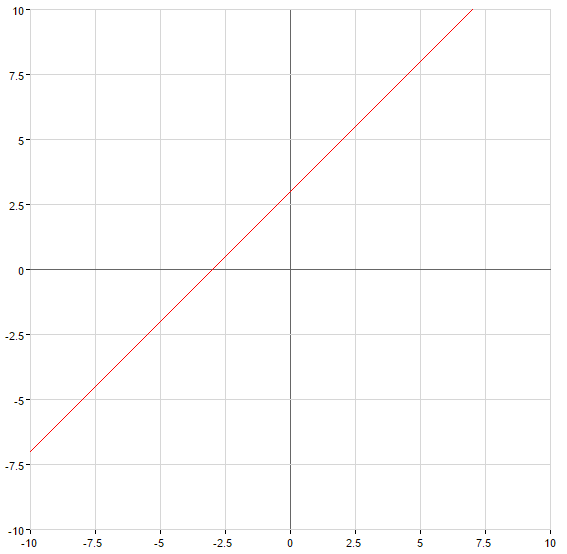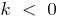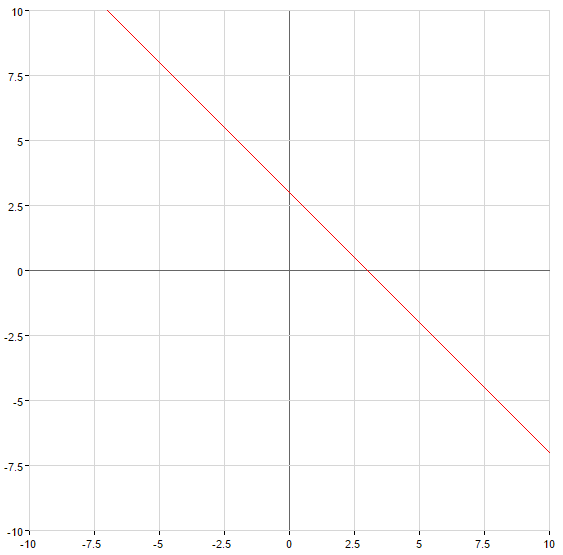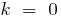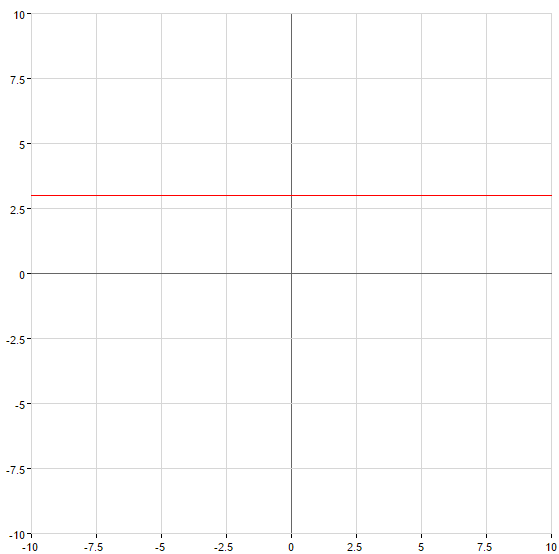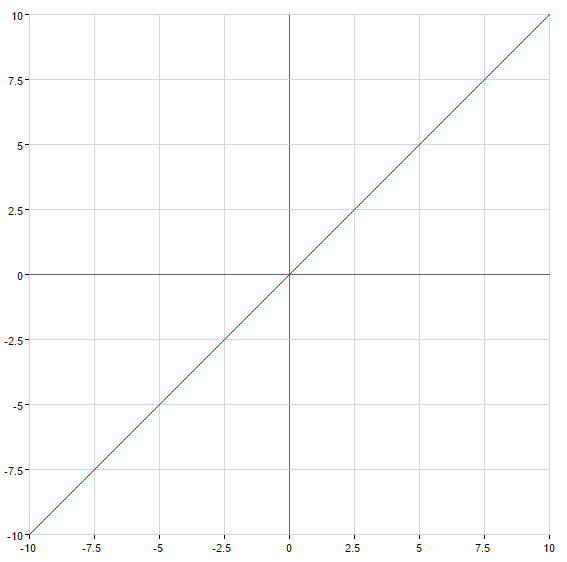Tags:
Chapter:
Versions in other languages:
Share with friends: